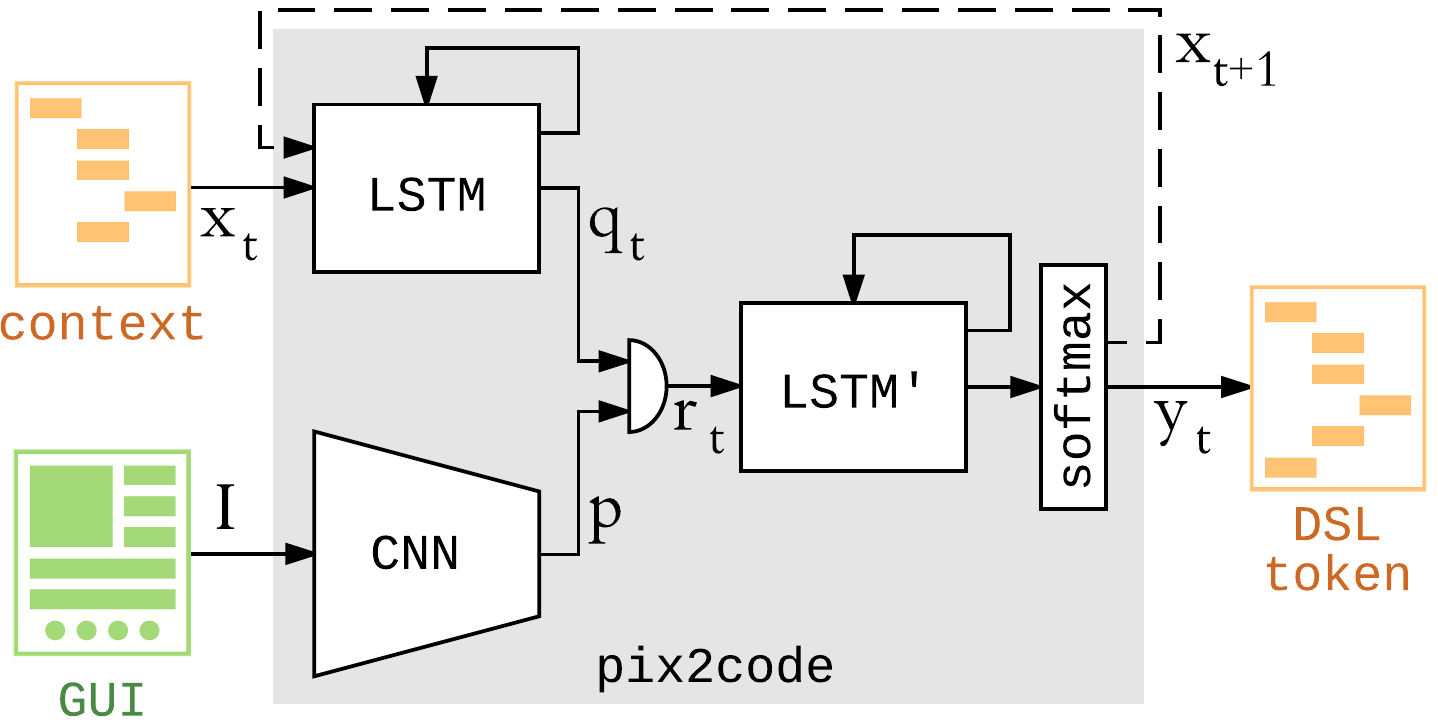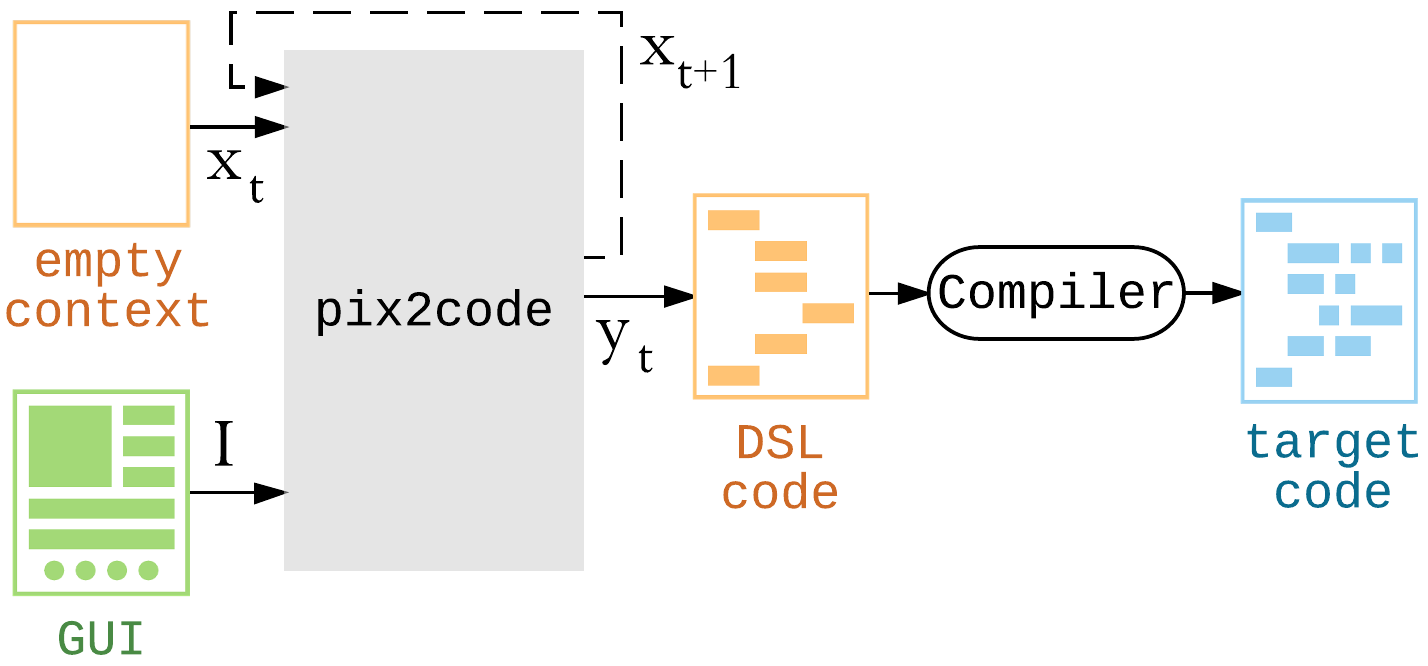## # pix2code: Generate Code from a Graphical User Interface Screenshot

similar to a image caption task

easy to create a lot of training data

use DSL as a smaller hypothsis space, one hot encode DSL tags## # Semi-supervised Knowledge Transfer for Deep Learning from Private Training Data

teachers trained on disjoint private data

students learn to predict by noisy voting from the teachers

model overfit and memorize data, attackers use hill-climbing from output probability, reveal real faces from dataset

strengthen this ensemble method by limited number of teacher votes, andy pick only topmost vote, after adding random noise

differential privacy

privacy loss

moment accountant

## # Making Neural Programming Architectures Generalize via Recursion

$$s_t = f_{enc}(e_t, a_t)$$ $$h_t = f_{lstm}(s_t, p_t, h_{t-1})$$ $$r_t = f_{end}(h_t), p_{t+1} = f_{prog}(h_t), a_{t+1} = f_{arg}(h_t)$$

• : time-step

• : state

• : domain-specific encoder

• : environment slice

• : environment arguments

• : core module

• : hidden LSTM state

• : decode return probability :

## # Understanding Deep Learning requires rethinkng generization

regularization does not stop overfitting data with random labels

SGD has implicit regularization

capacity control

matrix factorization

parameters memorize the datasets

optimization on random labels

model fit on Gaussian noise

## # Using Morphological Knowledge in Open-Vocabulary Neural Language Models

mixture model, with

• word model with finite vocabulary

• char based model, dealing with UNK and subword structures

• morphs model with max pooling on multiple morphes vectors, marginalize on multiple sequences of abstract morphemes

$$p(w_i \vert h_i) = \sum_{m_i = 1}^M p(w_i, m_i \vert h_i)$$ $$= \sum_{m_i=1}^M p(m_i \vert h_i)p(w_i \vert h_i, m_i)$$

as seen above, models are weighted to compute the probability of . The model assume that the selection of model i is conditionally independent of all models before it, given the sequence of word generated.

three word embeddings are concatednated and used as inputs, char embedding captures dative like Obame in Russian fro Obama; morphmes embeddings are max-pooled when different structures are find(say does can be decomposed as do+3-person+singular, or doe+plural)

## # Learning to Map sentences to Logical Form: Structured Classification with Probabilistic Categorial Grammers

• constants: map entities, numbers, or functions to a value

• logical connectors:

• conjunction
• disjunction
• negative
• implication
• quantification

• universal, any
• existential
• count
• argmax
• argmin
• definite l(lambda) reutrn the unique set for which the lambda exp is true
• lambda exp

### # CGG(combinatory categorial grammer)

1. functional application rules a/b b = a b a\b = a

2. functional application rules with semantics

### # MEASURING THE INTRINSIC DIMENSION OF OBJECTIVE LANDSCAPES

1. theta^D = theta_0^D + P theta^d
2. invariant to model width and depth, if fc layers
3. 90% performance as a threshold for intrinsic dimension
4. for RL task, performance is defined as max attained mean evaluation reward
5. 750 dimension for MNIST, invariant for fc layer, variant for convolution layers(measured on shuffled labels)
6. dense / sparse / fastfood random matrix

### # ORDERED NEURONS

forget gate and input gate defined by binary sequence, trainable by counting

overlap of forget and input shows structure

### # FeUdal

(2) directional cosine similarity rewards,

(3) goals specified with respect to a learned representation

(4) dilated RNN.

### # Simese Network

learn feature from verification task(same or different images), then one-shot learning new category and test images, using max likelihood

### # UNDERSTANDING THE ASYMPTOTIC PERFORMANCE OF MODEL-BASED RL METHODS

optimal planning horizon can be over 100 steps

combining model-based and model-free

• augment a model- free method with a model for faster learning
• make up for the asymptotic deficiencies of a model-based method by transitioning to model-free.

action-conditional predictor plan-conditional predictor